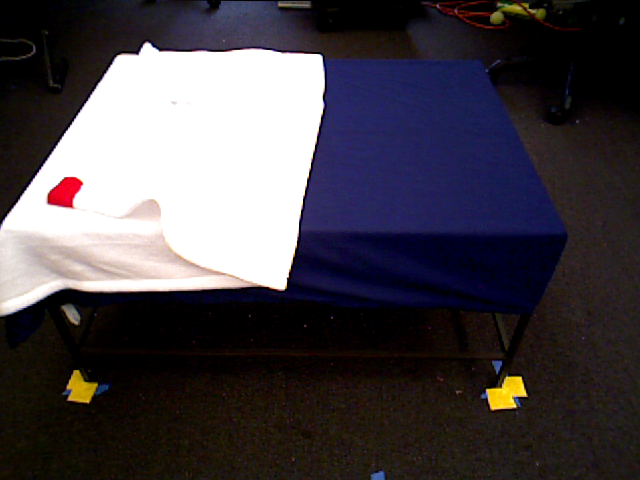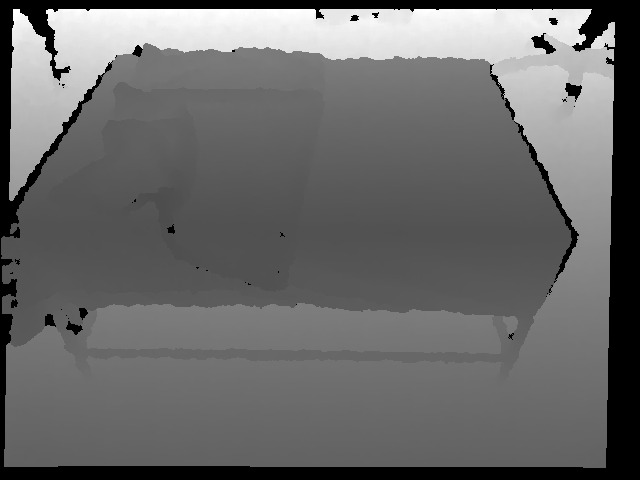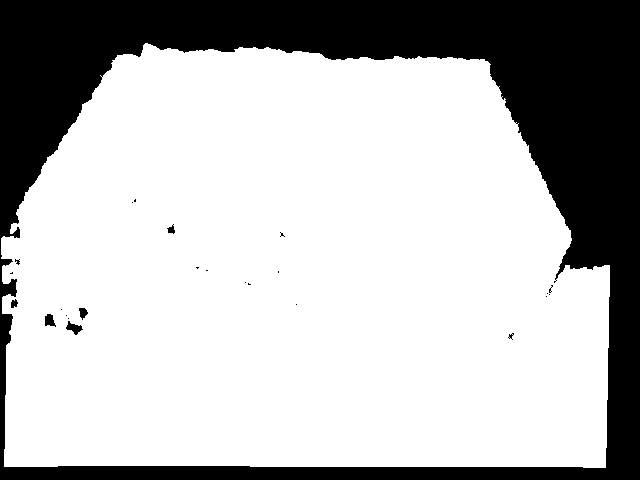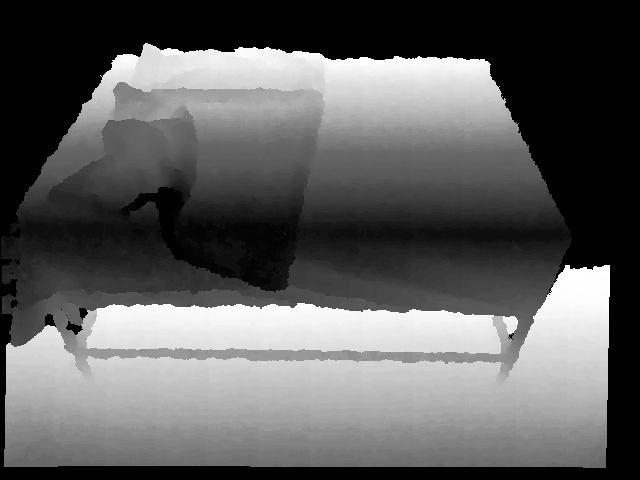Instantly share code, notes, and snippets.

#DanielTakeshi/processing depth images Last active Nov 13, 2018

Depth Image Processing
 """ For the BAIR Blog post. (c) 2018 by Daniel Seita (and Michael Laskey). """ import numpy as np import cv2 def depth_to_3ch(img, cutoff): """Useful to turn the background into black into the depth images. """ w,h = img.shape new_img = np.zeros([w,h,3]) img = img.flatten() img[img>cutoff] = 0.0 img = img.reshape([w,h]) for i in range(3): new_img[:,:,i] = img return new_img def depth_scaled_to_255(img): assert np.max(img) > 0.0 img = 255.0/np.max(img)*img img = np.array(img,dtype=np.uint8) for i in range(3): img[:,:,i] = cv2.equalizeHist(img[:,:,i]) return img def depth_to_net_dim(img): """Careful if the cutoff is in meters or millimeters! """ cutoff = 1400 img = depth_to_3ch(img, cutoff) cv2.imwrite('d_img_02.png', img) # all values above 255 turned to white img = depth_scaled_to_255(img) cv2.imwrite('d_img_03.png', img) # correct scaling to be in [0,255) now if __name__ == "__main__": d_img_raw = np.load('d_img_01.npy') cv2.imwrite('d_img_01.png', d_img_raw) depth_to_net_dim(d_img_raw)
Owner Author

### DanielTakeshi commented Oct 5, 2018

 Original RGB camera image:Original depth camera image (hard to see!), which I name `d_img_01`:`d_img_02`:`d_img_03`: